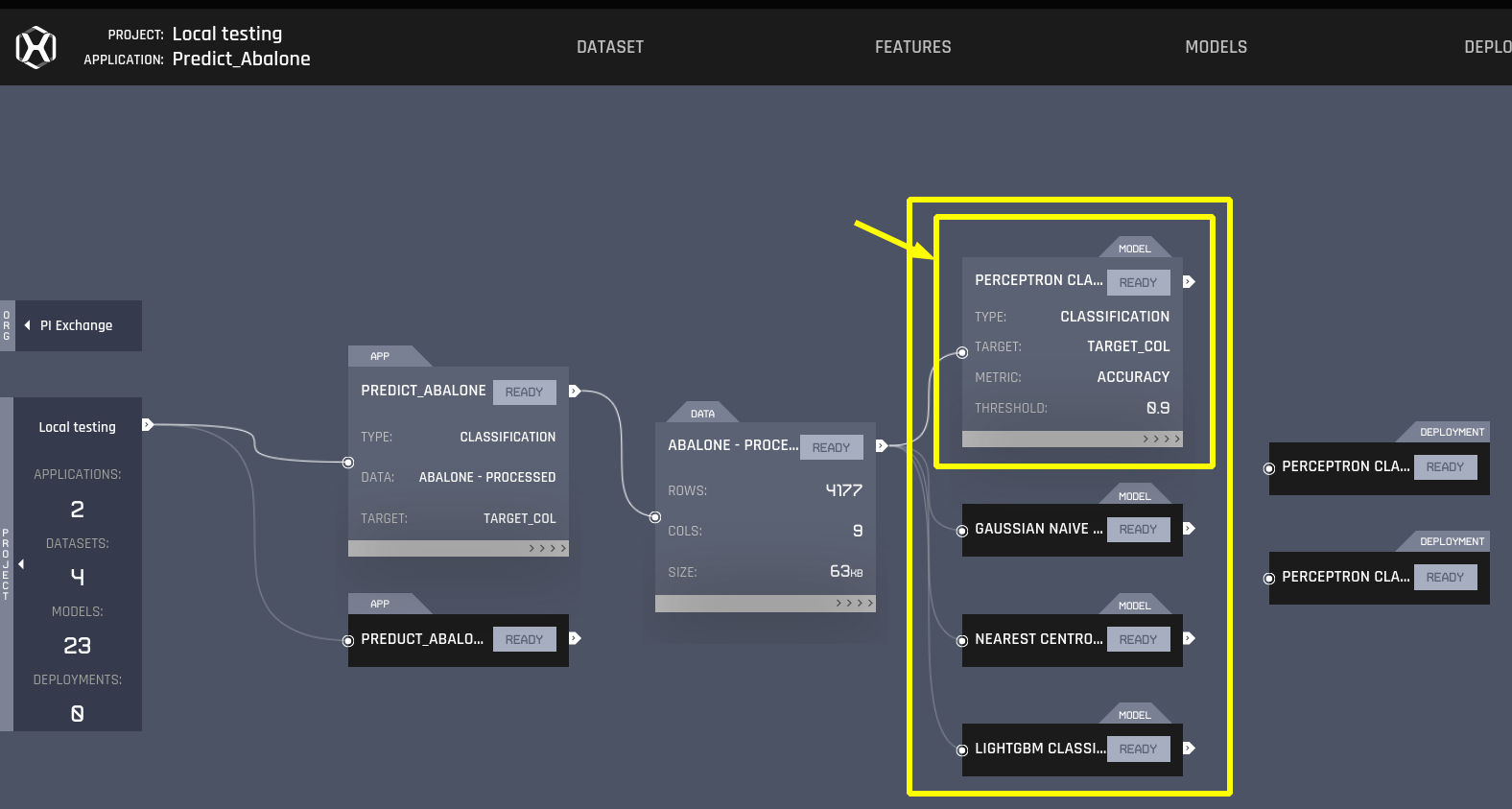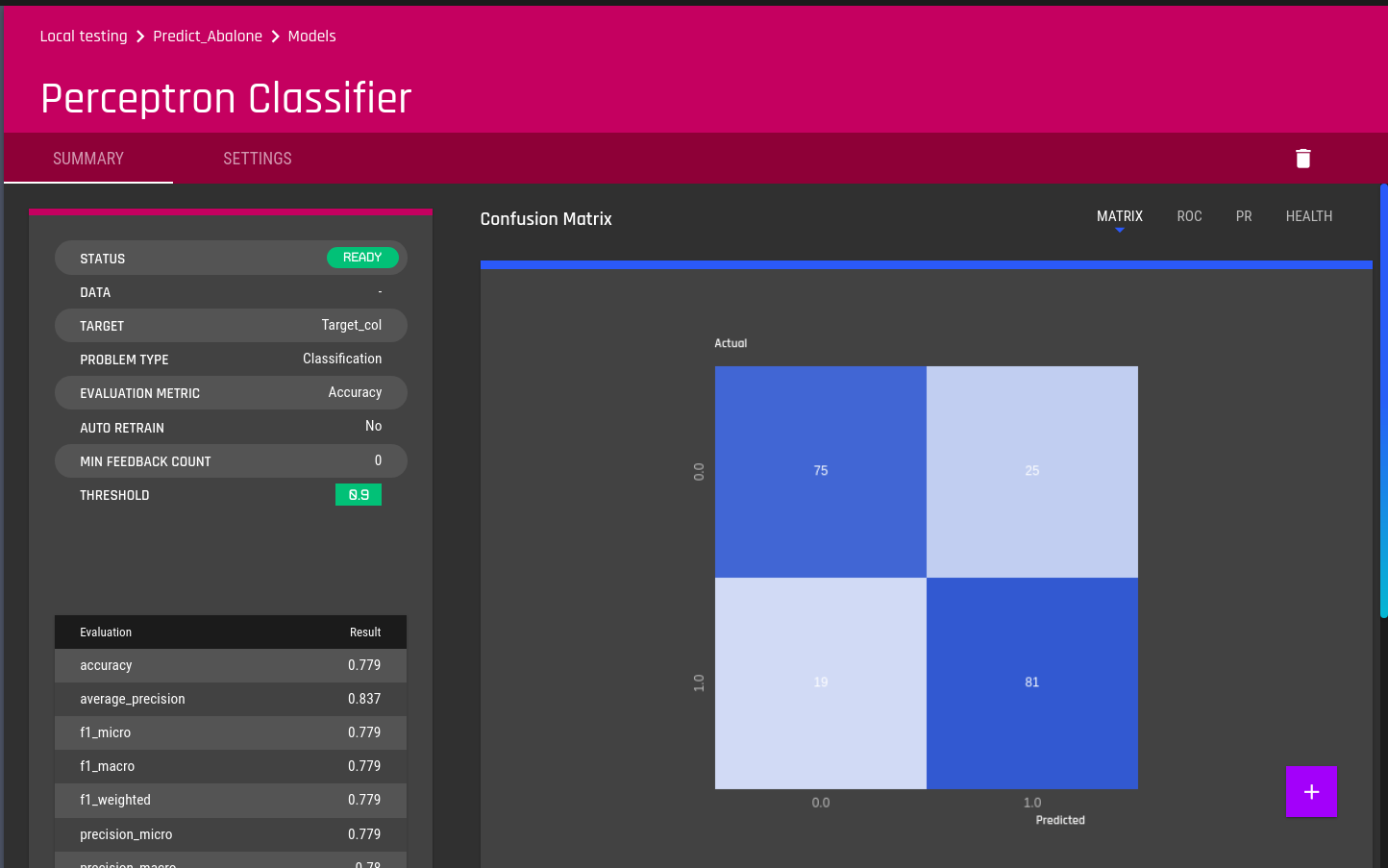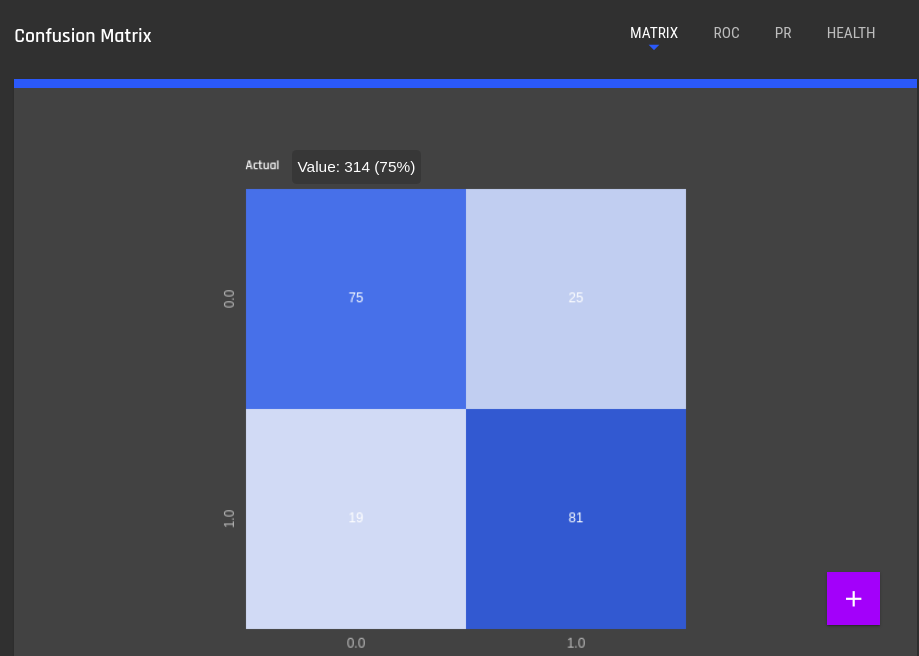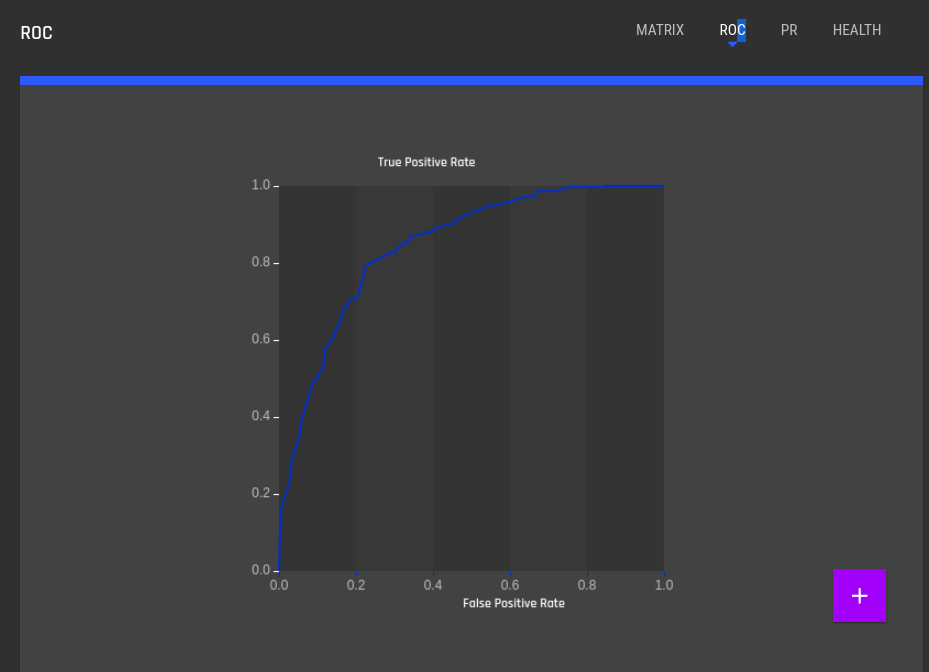# Obtaining Evaluation Results

This section describes how to obtain evaluation results of trained models in AI & Analytics Engine using

• Using the GUI
• Using API access through SDK

## Using the GUI

Obtaining the evaluation results per model is pretty straight forward. From the dashboard, as seen before, attached to the data rectangle there will be all the trained models. Select some sample model. (In the following example, the MLP classifier:Once inside the classifier, the training results are immediately displayed. On the left there's a summary of many "one line" metrics and the right side there are visual metrics: The confusion matrix, the ROC curve and PR curve.In this specific classification app, the relevant metrics are [accuracy, average precision, f1_micro, f1_macro, f1_weighted, ...]. The complete list is presented in the image below:Regarding the visuals, as explained, we are presented with the confusion matrix which allows us to easily count TP/FP/TN/FN values, the ROC curve (TPR vs FPR) and the precision-recall curve.## Using API access through SDK

To access the API functions, you need to first authenticate into the platform by the following

from aiaengine import api

client = api.Client()


### Import modules

Then you need to import the following modules that are used in the next steps.

import json

from aiaengine.api import model


### Obtain results

Now you can retrieve information of models. For those that are trained successfully, you can access the results of evaluation by the following

get_model_response = client.models.GetModel(
model.GetModelRequest(
id='id_of_model_to_explore'
)
)



The model_evaluation variable keeps the results over a range of evaluated metrics. The following is an example for classification models.

>>> model_evaluation['evaluation_scores']

{
'accuracy': 0.8530734632683659,
'average_precision': 0.6682887654106783,
'f1_micro': 0.8530734632683659,
'f1_macro': 0.739573077876545,
'f1_weighted': 0.8413883365361269,
'precision_micro': 0.8530734632683659,
'precision_macro': 0.7926047443268806,
'precision_weighted': 0.841784862340374,
'recall_micro': 0.8530734632683659,
'recall_macro': 0.7117236254200362,
'recall_weighted': 0.8530734632683659,
'roc_auc': 0.8463913814113215,
'prediction_time': 2.3829942938686906e-10,
'prediction_credits': 2.647771437631878e-12,
'train_time': 16.588364839553833,
'train_credits': 0.18431516488393146,
'predictive_performance': 73.9573077876545,
'f1_macro_rating': 3.9969590329172573,
'train_time_rating': 4.228687385582896,
'prediction_time_rating': 4.678588193150658,
'predictive_performance_rating': 3.9969590329172573
}